Next: Experiments Up: No Title Previous: Using Additional Information for

# Nonlinear Independent Factor Analysis

The nonlinear factor analysis model introduced in the previous section has Gaussian distributions for the sources. In this section we are going to show how that model can easily be extended to have mixture-of-Gaussians models for sources. In doing so we are largely following the method introduced in  for Bayesian linear independent factor analysis. The resulting model is a nonlinear counterpart of ICA or, more accurately, a nonlinear counterpart of independent factor analysis because the model includes finite noise. The difference between the models is similar to that between linear PCA and ICA because the first layer weight matrix A in the network has the same indeterminacies in nonlinear PCA as in linear PCA. The indeterminacy is discussed in the introductory chapter.

According to the model for the distribution of the sources, there are several Gaussian distributions and at each time instant, the source originates from one of them. Let us denote the index of the Gaussian from which the source si(t) originates by Mi(t). The model for the distribution for the ith source at time t is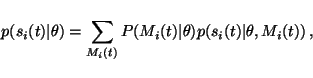(33)

whereis a time-independent Gaussian distribution with its own mean mij and log-std vij. The probabilities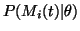of different Gaussians are modelled with time-independent soft-max distributions.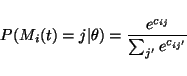(34)

Each combination of different Gaussians producing the sources can be considered a different model. The number of these models is enormous, of course, but their posterior distribution can still be approximated by a similar factorial approximation which is used for other variables.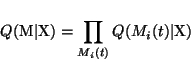(35)

Without losing any further generality, we can now write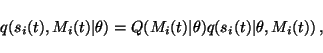(36)

which yields(37)

This means that the approximating ensemble for the sources has a form similar to the prior, i.e., an independent mixture of Gaussians, although the posterior mixture is different at different times.

Due to the assumption of factorial posterior distribution of the models, the cost function can be computed as easily as before. Let us denote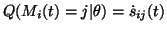and the posterior mean and variance ofby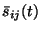and. It easy to see that the posterior mean and variance of si(t) are=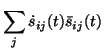(38)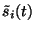=(39)

After having computed the posterior mean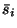and variance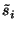of the sources, the computation of the Cp part of the cost function proceeds as with nonlinear factor analysis in the previous section. The Cq part yields terms of the form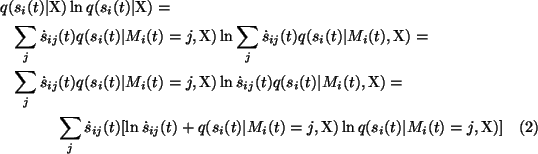and we have thus reduced the problem to a previously solved one. The terms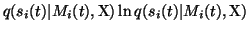are the same as for the nonlinear factor analysis and otherwise the equation has the same form as in model selection in Chap. 6. This is not surprising since the terms Q(Mi(t) | X) are the probabilities of different models and we are, in effect, therefore doing factorial model selection.

Most update rules are the same as for nonlinear factor analysis. Equations (39) and (40) bring the termsfor updating the means mij and log-std parameters vij of the sources. It turns out that they both will be weighted with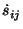, i.e., the observation is used for adapting the parameters in proportion to the posterior probability of that observation originating from that particular Gaussian distribution.Next: Experiments Up: No Title Previous: Using Additional Information for
Harri Lappalainen
2000-03-03